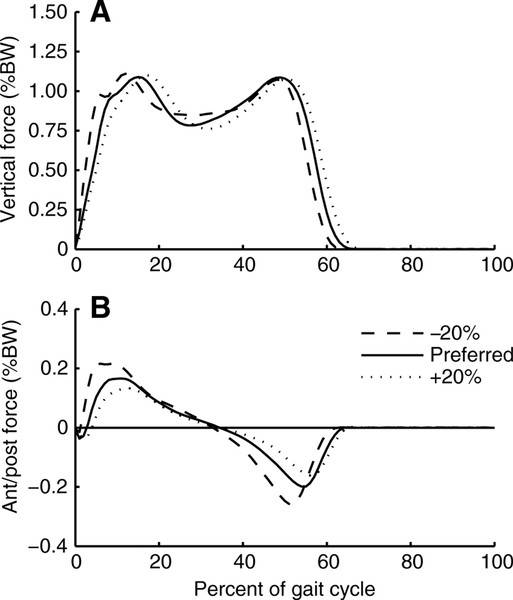# Friction's role in walking

In summary, friction only acts on the foot. It does not act at the center of mass, and any unbalanced force will cause the center of mass to accelerate.f

When we loose the touch with ground no friction is acting..so how we accelarate ? also friction prevent our foot from sliding but also accelarates our body ? how is it possible to acting in 2 different points ( foot and center of mass ) ?

When we loose the touch with ground no friction is acting..so how we accelarate ?
First, we never loose contact with the ground when walking, but when running during the time that both feet are off the ground the acceleration is downwards due to gravity.

also friction prevent our foot from sliding but also accelarates our body ? how is it possible to acting in 2 different points ( foot and center of mass ) ?
Friction only acts at the foot. It does not act at the center of mass. Any unbalanced force will cause the center of mass to accelerate. The unbalanced force does not need to act at the center of mass.

If there was not any friction on the ground, it would be slick and thus your feet could not grip the surface.

Motion occurs when the force applied by your legs at your feet overcomes the force of friction at the surface.

F=ma=Ffeet - Ffriction

Walking is the process of repeatedly falling over, catching yourself, then lifting yourself back up.

Motion occurs when the force applied by your legs at your feet overcomes the force of friction at the surface.
Motion occurs when there is non-zero friction at the surface and no opposing force. "Overcoming" friction generally means slipping.

Motion occurs when the force applied by your legs at your feet overcomes the force of friction at the surface.

F=ma=Ffeet - Ffriction
Hmm, this isn’t quite right. There is no force of friction to overcome. The force of friction is the force that propels you forward in normal walking. You don’t overcome it, you use it.

•jbriggs444
So then what would the equation be? I was thinking about it above like pushing or pulling a block.

As the foot pushes, it is acting slightly in reverse so friction is in the forward direction. The push on the surface has an equal and opposite.

F=ma=Ffriction + Fsurface

So then what would the equation be? I was thinking about it above like pushing or pulling a block.

As the foot pushes, it is acting slightly in reverse so friction is in the forward direction. The push on the surface has an equal and opposite.

F=ma=Ffriction + Fsurface
What is Ffriction? On what body does it act? What is Fsurface? On what body does it act?

I was implying that it acted on the feet of the walker.

I was implying that it acted on the feet of the walker.

Newton's third law (the action and reaction thing) speaks of force-pairs acting on different objects. The "action" force acts on one object and the "reaction" force acts on another. For example, the foot pushes on the ground and the ground pushes on the foot.

Newton's second law (∑F=ma) speaks of the various forces acting on the same object.

If you have a action-reaction force pair from the third law (hence two objects) you should probably not be invoking the second law and adding the two individual forces together because they do not act on the same object.

Last edited:
So then what would the equation be? I was thinking about it above like pushing or pulling a block.
So, if you are pushing a block then there will be 4 forces acting on you. Gravity, the normal force on your feet, friction on your feet, and the normal force on your hands.

•osilmag
So then what would the equation be?
Ignoring air resistance:

ma = Ffriction + Fnormal

Along the direction of walking Ffriction points backward in the early stance, and forward during late stance (push off) (Figure B). At constant walk speed the impulse from friction must cancel over a walk cycle:From: http://jeb.biologists.org/content/210/18/3255

#### Attachments

•osilmag, russ_watters and Dale